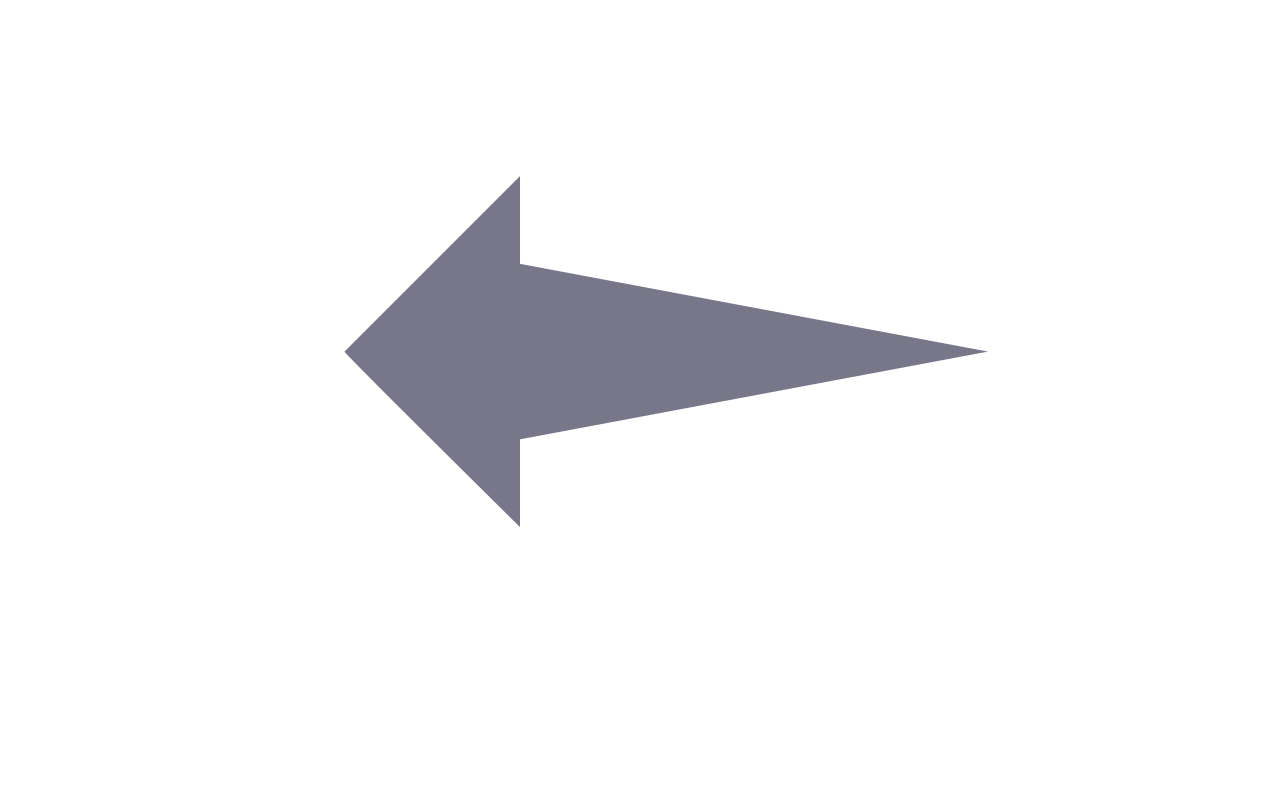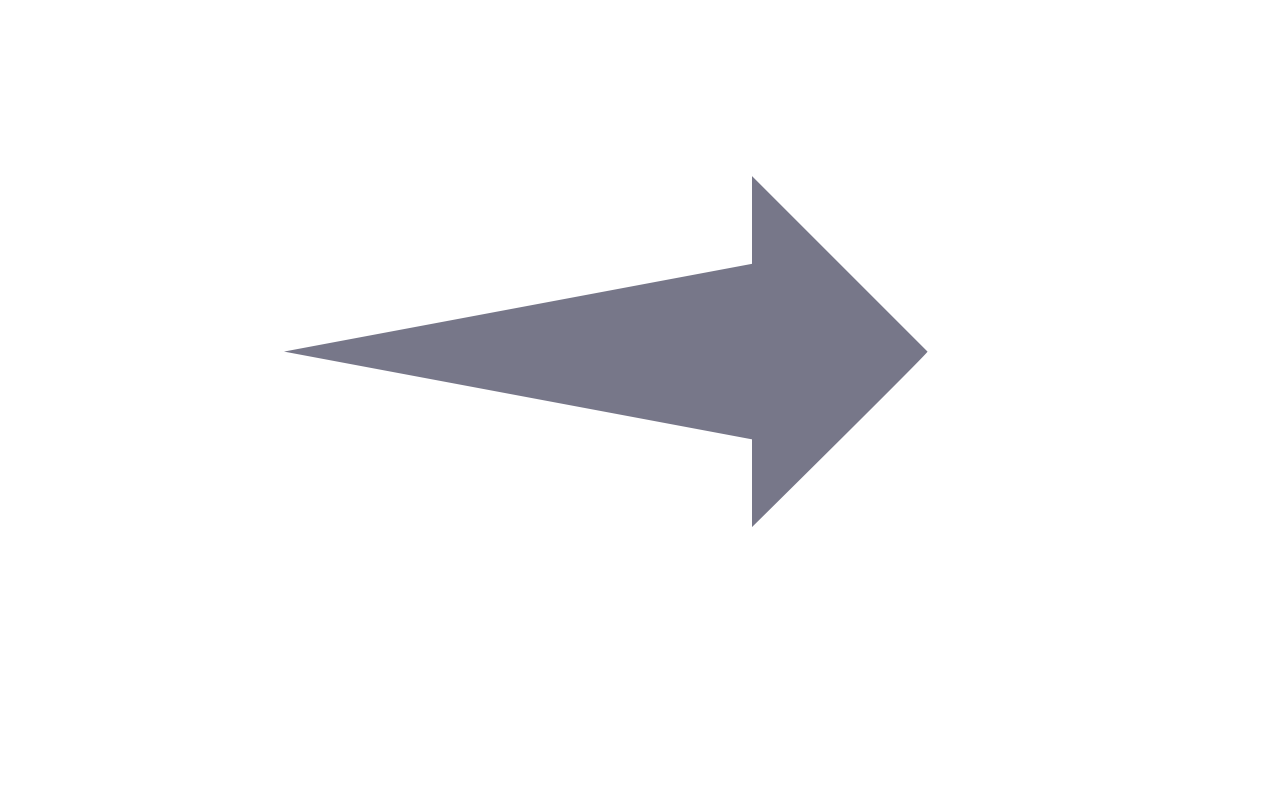### Explaining Multi-stage Tasks by Learning Temporal Logic Formulas from Suboptimal Demonstrations

Glen Chou, Necmiye Ozay, Dmitry Berenson

### Abstract

We present a method for learning to perform multi-stage tasks from demonstrations by learning the logical structure and atomic propositions of a consistent linear temporal logic (LTL) formula. The learner is given successful but potentially suboptimal demonstrations, where the demonstrator is optimizing a cost function while satisfying the LTL formula, and the cost function is uncertain to the learner. Our algorithm uses the Karush-Kuhn-Tucker (KKT) optimality conditions of the demonstrations together with a counterexample-guided falsification strategy to learn the atomic proposition parameters and logical structure of the LTL formula, respectively. We provide theoretical guarantees on the conservativeness of the recovered atomic proposition sets, as well as completeness in the search for finding an LTL formula consistent with the demonstrations. We evaluate our method on high-dimensional nonlinear systems by learning LTL formulas explaining multi-stage tasks on 7-DOF arm and quadrotor systems and show that it outperforms competing methods for learning LTL formulas from positive examples.

### Live Paper Discussion InformationStart Time End Time
07/16 15:00 UTC 07/16 17:00 UTC

### Paper Reviews

Review 2

This paper studies the problem of learning multi-stage tasks given a set of positive demonstrations from an approximately optimal demonstrator. Specifically the paper studies a variant of Apprenticeship Learning/ Inverse Reinforcement Learning (IRL) where parts of the task are described by a fragment of Linear Temporal Logic. This follows a growing body of literature studying similar problems to address the fact traditional IRL (which learns rewards) does not easily extend to sequential tasks. The approach taken in this paper is at a high level straightforward, but technically impressive. The authors propose: 1. Parameterizing the space of cost functions and specifications. 2. Searching for parameters that "explain" the demonstrations. However, like tradtional IRL - or perhaps more so - the notion of "explains" is highly degenerate. To alleviate this, the authors propose searching for parameters that make the demonstrator "approximately-optimal" where optimality is independently evaluated both at level of low level control and with respect to the specification. The low level critera optimality critera is given as the KKT conditions, which is never fully justified. I must admit, that while thorough, the exposition in this section was quite terse and at some points dizzying. Even being somewhat familar with the embedding of temporal logic constraints as Mixed Interger Constraints, I found this part hard to follow. The specification optimality critera later comes in the form of "minimality" in the atomic propositions "visited". While I'm not certain, believe that this minimality might correspond to the path length in the monitoring buchi-automata, subject to dynamic feasbility. The ultimate algorithm resembles counter example guided inductive synthesis. The essential idea is to check if there exists a formula with a bounded syntatic dag size that is approximately optimal - where the "approximate" comes in the form of a meta-parameter bounding the distance from optimality. The refuted formula are used to synthesize counter example trajectories which serve to tighten the concept class. If no formula of a particular DAG size exists, the DAG size is increased. This length based search, which uses a previously establish SAT encoding of possible parse trees, is both systematic and serves to regularize the learner, since larger formulas are more expressive - and thus easier to overfit. Finally, the paper evaluates this technique on a number of impressive examples and demonstrates that the addition of goal directed behavior does indeed output perform a similar techniques for learning from positive examples. My primary concerns are: 1. How does this algorithm deal uncertainty in the dynamics. The approximate KKL satisfaction seems like a potential solution, but doesn't explicitly model agents accounting for risk. 2. Is there a way to measure how "confident" the algorithm is in the returned result. 3. Is there a way to robustify this algorithm to mis-labeled or unlabeled examples. In particular, it seems to me that incorrectly refuting a trajectory in step 10 of Alg 1 could have disastrous consequences for learning algorithm, despite ample evidence in the rest of the demonstrations.